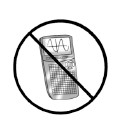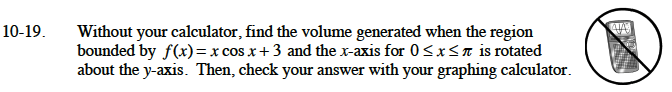Home > CALC > Chapter Ch10 > Lesson 10.1.3 > Problem10-19

10-19.Without your calculator, find the volume generated when the region bounded by f(x) = x cos x + 3 and the x-axis for 0 ≤ x π is rotated about the y-axis. Then, check your answer with your graphing calculator. Homework Help ✎Use shells:

$\int_0^\text{?}2\pi x(f(x))dx$

You will need to use integration by parts twice.